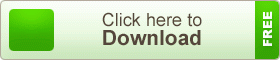# SSC Higher Mathematics Note 5th Chapter Equations

SSC Higher Mathematics Note 5th Chapter Equations. It has been before that unknown quantities or variables are very important in Algebra. In practical life, we use xyetc. as symbols to represent the unspecified object, number or objects. These symbols are called variables or unknown quantities. An expression is formed with more than one unknown quantity or variable; such as 2yx2 + z+ 2etc. When an unknown quantity or expression is equated to a definite number or value, then it is called an equation. The equation is a very important topic in Algebra. A lot of practical problems can be solved using equations.

## SSC Higher Mathematics Note 5th Chapter EquationsLinear and quadratic equations in one variable and linear equations in two variables have been discussed in details in Secondary Algebra Book. A quadratic equation in one variable can be solved easily after factorizing the left-hand side of the equation if the roots are rational. But all expressions cannot be factorized easily. That is why the following procedure is used to solve the quadratic equation of any form.

teachingbd24.com is such a website where you will get all kinds of necessary information regarding educational notes, suggestions and question patterns of schools, colleges, and madrasas. Particularly, you will get here special notes of physics that will be immensely useful to both students and teachers. The builder of the website is Mr. Md. Shah Jamal who has been serving for 33 years as an Assistant Professor of Physics at BAF Shaheen College Dhaka. He expects that this website will meet up all the needs of Bengali version learners /students. He has requested concerned students and teachers to spread this website home and abroad.

SSC Higher Mathematics Note